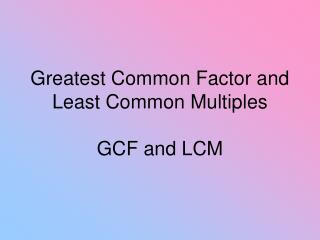# Greatest Common Factor and Least Common Multiples GCF and LCM - PowerPoint PPT PresentationDownload PresentationGreatest Common Factor and Least Common Multiples GCF and LCM

Greatest Common Factor and Least Common Multiples GCF and LCMDownload Presentation## Greatest Common Factor and Least Common Multiples GCF and LCM

- - - - - - - - - - - - - - - - - - - - - - - - - - - E N D - - - - - - - - - - - - - - - - - - - - - - - - - - -
##### Presentation Transcript

1. Greatest Common Factor and Least Common MultiplesGCF and LCM

2. What is the difference between a factor and a multiple?

3. Give me an example of a factor of 15

4. Give me an example of a multiple of 15

5. How would you find the GCF of 60 and 96?

6. There are actually 3 ways. You can use prime factorization, write out all the prime factors for each number or extract common prime numbers.

7. List the factors of 60:1,2,3,4,5,6,10,12,15,20,30,60List the factors of 961,2,3,4,6,8,12,16,24,32,48,96find the largest factor - 12

8. …or do prime factorization. Circle all the primes the 2 numbers have in common and multiply one set of them to get your GCF. 96 60 48 2 2 30 24 2 15 2 12 2 3 5 6 2 2 x 2 x 3 = 12 2 3

9. …or you can extract the common prime factors of 60 and 96 and form a product to give the GCF. This, to me, is the easiest way. Try it! 2 60, 96 2 x 2 x 3 = 12 2 30, 48 3 15, 24 5, 8

10. Find the GCF (HCF) of 36, 24, 144 and 96

11. 96 24 36 48 2 2 12 18 2 24 2 6 9 2 2 12 2 3 2 3 3 6 2 144 2 3 12 12 3 4 2 x 2 x 3 = 12 3 4 2 2 2 2

12. 2 36, 24, 144, 96 2 x 2 x 3 = 12 2 18, 12, 72, 48 3 9, 6, 36, 24 3 2 12 8

13. There are 3 ways to find the LCM as well. You can list the multiples of the numbers, do prime factorization, or extract the prime factors from either one or both numbers.Find the LCM of 12 and 18

14. Multiples of 12 are…12,24,36,48,60,72,….Multiples of 18 are…18,36,54,72,90,108,…The smallest multiple the 2 numbers have in common is the least common multiple.

15. …or do prime factorization. Write down the number they have in common only once, then write down the leftover numbers. Multiply them all together. 12 18 9 4 2 3 3 3 2 2 Numbers in common are 2 and 3 Leftover numbers are 2 and 3 2 x 3 x 2 x 3 = 36

16. Remember…the numbers you pull out have to be prime numbers! 2 12, 18 2 6, 9 3 3, 9 3 1, 3 1 1 2 x 2 x 3 x 3 = 36

17. Find the LCM of 35, 420 and 245

18. 245 35 420 42 5 49 10 5 7 5 7 7 2 6 7 2 3 Numbers they have in common: 5 and 7 Leftover numbers: 2, 3, 2, 7 Multiply them all together: 5 x 7 x 2 x 3 x 2 x 7 = 2940

19. primes 5 35, 420, 245 7 7, 84, 49 7 1, 12, 7 3 1, 12, 1 2 1, 4, 1 2 1, 2, 1 1, 1, 1 5 x 7 x 7 x 3 x 2 x 2 = 2940

20. Find the GCF and LCM of 28 and 63

21. 7 28 63 4 9 GCF = 7 LCM = 7 x 4 x 9 = 252

22. Find the GCF and LCM of12 and 13

23. 1 12 13 GCF = 1 LCM = 1 x 12 x 13 = 156

24. Find the GCF and LCM of 27, 21, 33 and 15

25. 3 27 21 33 15 9 7 11 5 GCF = 3 LCM = 3 x 9 x 7 x 11 x 5 = 10,395

26. Start tonight’s homework. No calculators!